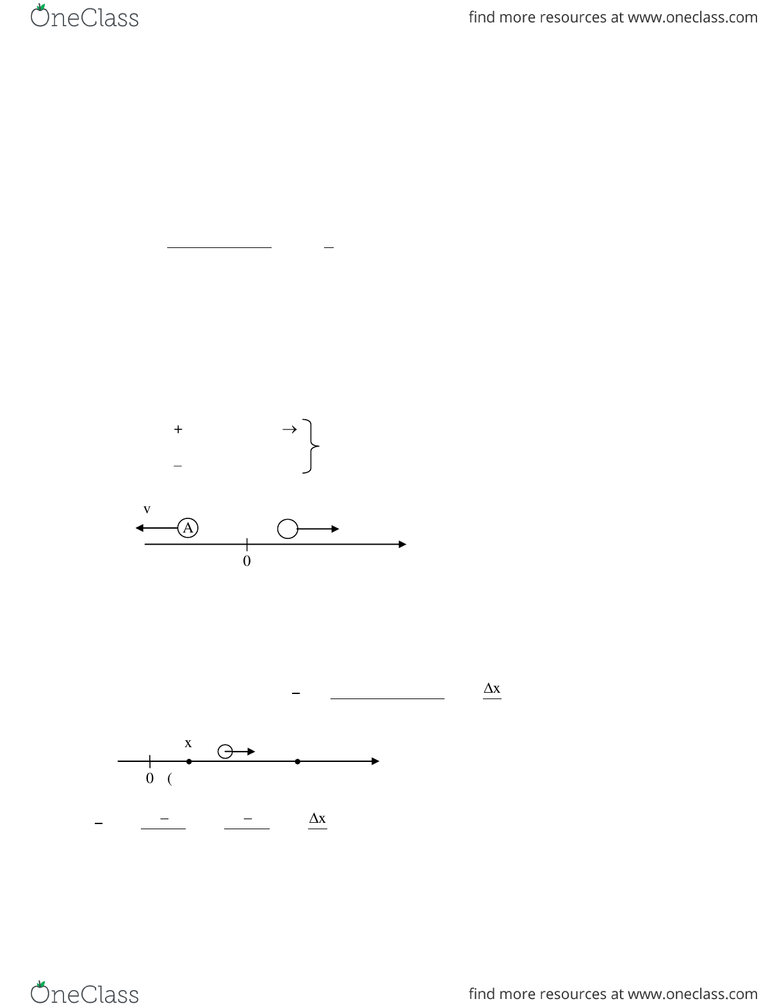Class Notes (1,100,000)
US (480,000)
CU-Boulder (3,000)
PHYS (100)
Lecture 1

# PHYS 1110 Lecture 1: 02_Motion1D

Department
Physics
Course Code
PHYS 1110
Professor
prof.stevenpollock
Lecture
1

Page:
of 161D - 1
9/28/2013 Dubson Notes University of Colorado at Boulder
Motion in one dimension (1D)
In this chapter, we study speed, velocity, and acceleration for motion in one-dimension. One
dimensional motion is motion along a straight line, like the motion of a glider on an airtrack.
speed and velocity
distance traveled d
speed , s = , units are m/s or mph or km/hr or...
time elapsed t
speed s and distance d are both always positive quantities, by definition.
velocity = speed + direction of motion
Things that have both a magnitude and a direction are called vectors. More on vectors in Ch.3.
For 1D motion (motion along a straight line, like on an air track), we can represent the direction
of motion with a +/ sign
Objects A and B have the same speed s = |v| = +10 m/s, but they have different velocities.
If the velocity of an object varies over time, then we must distinguish between the average
velocity during a time interval and the instantaneous velocity at a particular time.
Definition:
change in position x
average velocity = v change in time t

f i 2 1
f i 2 1
x x x x x
vt t t t t

 
 
x = xfinal xinitial = displacement (can be + or )
+ = going right
= going left
always!
B
vB = +10 m/s
vA = 10 m/s
A
x
(final)
(initial)
x
0
x1
x2
1D - 2
9/28/2013 Dubson Notes University of Colorado at Boulder
Notice that (delta) always means "final minus initial".
x
vt
is the slope of a graph of x vs. t
Review: Slope of a line
Suppose we travel along the x-axis, in the positive direction, at constant velocity v:
x
0
start
x
x
t
x2
t
x1
t1
t2
y
x
slope =
rise
run
=
=
x
t
= v
y-axis is x, x-axis is t .
x
y
y
x
y
x
slope =
rise
run
=
x
y
(+) slope
x
y
() slope
x
y
0 slope
y2 y1
=
x2 x1
(x1, y1)
(x2, y2)
1D - 3
9/28/2013 Dubson Notes University of Colorado at Boulder
Now, let us travel in the negative direction, to the left, at constant velocity.
Note that v = constant slope of x vs. t = constant graph of x vs. t is a straight line
But what if v constant? If an object starts out going fast, but then slows down and stops...
The slope at a point on the x vs. t curve is the instantaneous velocity at that point.
Definition: instantaneous velocity = velocity averaged over a very, very short (infinitesimal)
time interval
t0
x d x
v lim t d t


= slope of tangent line. In Calculus class, we would say that the
velocity is the derivative of the position with respect to time. The derivative of a function x(t) is
defined as the slope of the tangent line:
t0
d x x
lim
d t t

.
x
x
t
t
x
t
x
start
x
x < 0
t
t
slope = v =
x
t
< 0
x
slower
slope > 0 (fast)
t
slope = 0 (stopped)